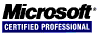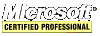# PHP Quick Tutorial

## Constants

Constants are often used in functions that require specific values to be passed in. Use the `define` function to create constants.

``````<?php
define("MAX", 1000);
define("TRUE_MAX", MAX);

echo MAX;
echo TRUE_MAX;
?>
``````

A reference is a variable that points to another variable. Changing one changes both (or all that are referenced).

``````<?php
\$i = 321;
\$j = &\$i; // \$j is a reference to \$i

echo \$i;
echo \$j;

\$i = 654;

echo \$i;
echo \$j;

\$j = 987;

echo \$i;
echo \$j;
?>
``````

## Operators

There are operators in PHP to handle arithmetic, assignment, comparison, etc.

operatoroperation
=assigns right operand to the left operand
==is true when the two operands are equal
!=is true when the two operands are not equal
===is true when the two operands are identical
!==is true when the two operands are not identical
<is true when left operand is less than right operand
>is true when left operand is greater than right operand
<=is true when left operand is less than or equal to right operand
>=is true when left operand is greater than or equal to right operand
&&is true when both left and right operands are true
||is true when either left or right operand is true
++increment operand by one
--decrement operand by one
+=increments left operand by right operand
-=decrements left operand by right operand
.concatenates left and right operand
%divides the left operand by the right and returns the remainder
|bitwise OR: returns the bits that are on in either the left or right operands
&bitwise AND: returns the bits that are one both in the left and right operands
?:ternary operator is like a condensed conditional statement
`execution operator (the backtick key)

An example using the execution operator:

``````<?php
\$output = `ls -al`;
echo '<pre>';
echo \$output;
echo '</pre>';
?>
``````

## Conditional Statement

A conditional statement takes a different action depending on the outcome of a test. Any number that is not zero is considered `true` so zero is consider `false`. Empty strings evaluate to `false`. The `===` operator compares values and data types, if they are the same data type with the same data then they are identical.

``````<?php
\$i = 10;

if (\$i) {
echo '\$i is not 0';
}

if(10 == "10") { // this is true
echo "EQUAL";
}

if(10 === "10") { // this is not true
echo "IDENTICAL";
}

if (\$i == 0) {
echo 'zero';
}
else if (\$i < 5) {
echo 'five';
}
else if (\$i >= 100 && \$i < 200) {
echo 'huge';
}
else if (\$i <= 10) {
echo 'ten';
}
?>
``````

## Switch Statement

Sometimes a `switch` is more appropriate for executing code based on conditions.

``````<?php
\$animal = 'dog';

switch(\$animal) {
case 'cat':
echo 0;
break;
case 'pony':
echo 1;
break;
case 'snake':
echo 2;
break;
case 'dog':
echo 3;
break;
default:
echo 10;
}
?>
``````

## Loop Statements

There are four ways to execute a block of code multiple times. These are with the `while`, `for`, `foreach`, and `do while` loop statements.

The `while` loop executes a block of code for as long as a given condition is true.

``````<?php
\$i = 0;
while(\$i < 10) {
echo \$i;
\$i = \$i + 1;
}
?>
``````

The `for` loop specifies a declaration, a condition, and an action.

``````<?php
for(\$i = 0; \$i < 10; \$i = \$i + 1) {
echo \$i;
}
?>
``````

The `foreach` loop is specifically for objects and arrays.

``````<?php
\$arr = array(1, 2, 3, 4, 5);
foreach(\$arr as \$i) {
echo \$i;
}
?>
``````

The `foreach` loop can access the keys and values of the array.

``````<?php
\$arr = array(5, 4, 3, 2, 1);
foreach(\$arr as \$key => \$value) {
echo "[\$key] is set to \$value\n";
}
?>
``````

The `do while` loop is like the `while` one except the condition is evaluated at the end of the code block.

``````<?php
\$i = 0;
do {
echo \$i;
\$i = \$i + 1;
} while(\$i < 10);
?>
``````

## exit

Use the `exit` construct to terminate the processing of the PHP script file.

``````<?php
exit;
echo 'THIS WILL NOT BE OUTPUT!';
?>
``````
<< < [Page 2 of 4] > >>